# Final Project Documentation

### Testing sensors

1. HCHO (VOC) sensor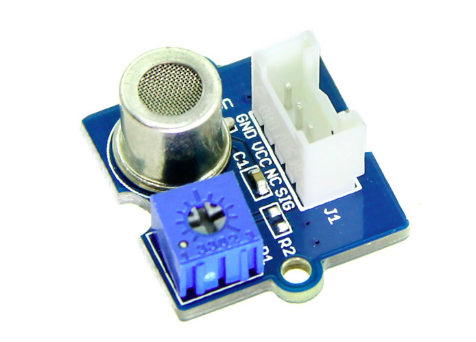For Data reading of VOC sensor I could use the manufacturer code as to see below. First Code shows the Part 1 of the programming the sensor, which is the calibrating part.
```
demo of Grove - HCHO Sensor by seeds studio

#include              // load the math.h library

#define Vc 4.95

#define R0 34.28              // the number of R0 you detected just now

void setup()                  // At the begining of the programm connect to the serial port
{
Serial.begin(9600);
}

void loop()
{
int sensorValue=analogRead(A1);     // read the signal (voltage value) from the pin A1 (analog)
double Rs=(1023.0/sensorValue)-1;   // calculate Rs out of the read voltage value of pin A1
Serial.print("Rs = ");
Serial.println(Rs);                 // Print the real value of Rs
double ppm=pow(10.0,((log10(Rs/R0)-0.0827)/(-0.4807)));  // calculate the HCHO level withs this callculation (ppm)
Serial.print("HCHO ppm = ");        // Print the VOC value
Serial.println(ppm);
delay(10000);                        //wait 10 seconds bevor you read the next value
}

```

After the calibration, when I was sitting in the garden, there was a car nearby that started the engine. Immediately, even if I did not think about it, the sensor data has risen enormously. A few minutes later, a neighbor started the barbecue and the sensor data went up immediately ...

After the calibration in the outside air, I could bring the sensor into the room and measure the data of the room air. The sensor data kept rising as I was in the room with the door closed and after opening the window, the value of HCHO quickly dropped off.

2. Temperature sensor

Grove temperature sensor v1.2 Seeds Studio .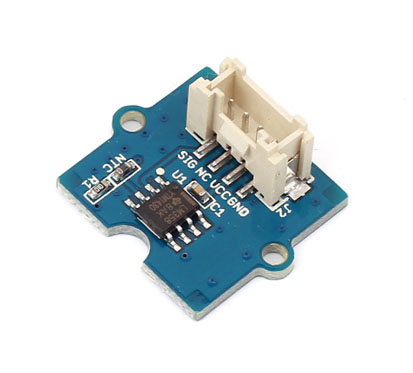Here you can find the simple temperature and HCHO data reading code:
```// Demo code for Grove - Temperature Sensor V1.1/1.2
// Loovee @ 2015-8-26

#include

// Following two constants belonge to the HCHO sensor:

#define Vc 4.95
#define R0 34.28                  //the number of R0 you detected during the calibration

const int B = 4275;               // B value of the thermistor
const int Rinit = 100000;         // Rinit = 100k
const int pinTempSensor = A0;     // temperature sensor connect to A0

void setup()
{
Serial.begin(9600);
}

void loop()
{

float R = 1023.0/a-1.0;
R = Rinit*R;

float temperature = 1.0/(log(R/Rinit)/B+1/298.15)-273.15 - 2; // convert to temperature via datasheet

int sensorValue=analogRead(A1);     // read the signal (voltage value) from the pin A1 (analog)
double Rs=(1023.0/sensorValue)-1;   // calculate Rs out of the read voltage value of pin A1

Serial.print("Rs = ");
Serial.println(Rs);                 // Print the real value of Rs
double ppm=pow(10.0,((log10(Rs/R0)-0.0827)/(-0.4807)));  // calculate the HCHO level withs this callculation (ppm)

Serial.print("HCHO ppm = ");        // Print the VOC value
Serial.println(ppm);

Serial.print("temperature (degrees Celsius) = ");
Serial.println(temperature);

delay(2000);
}```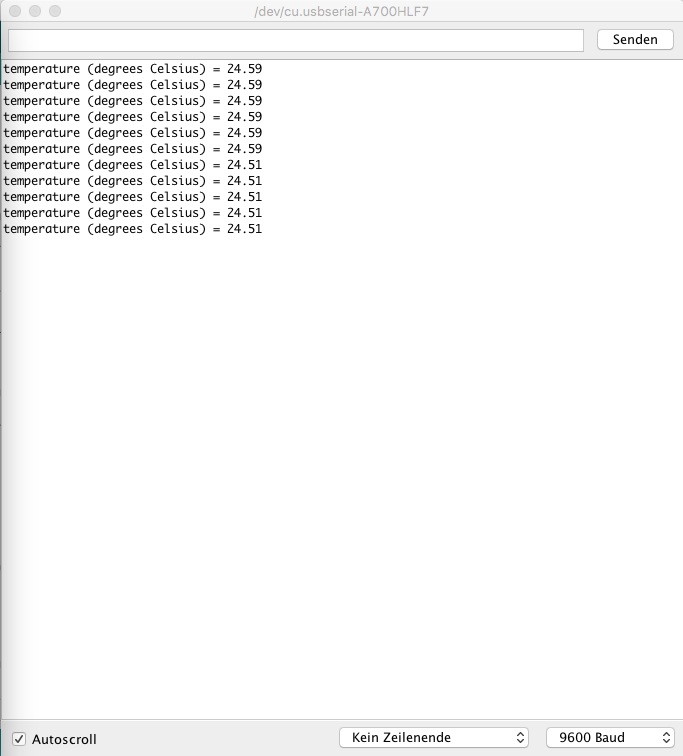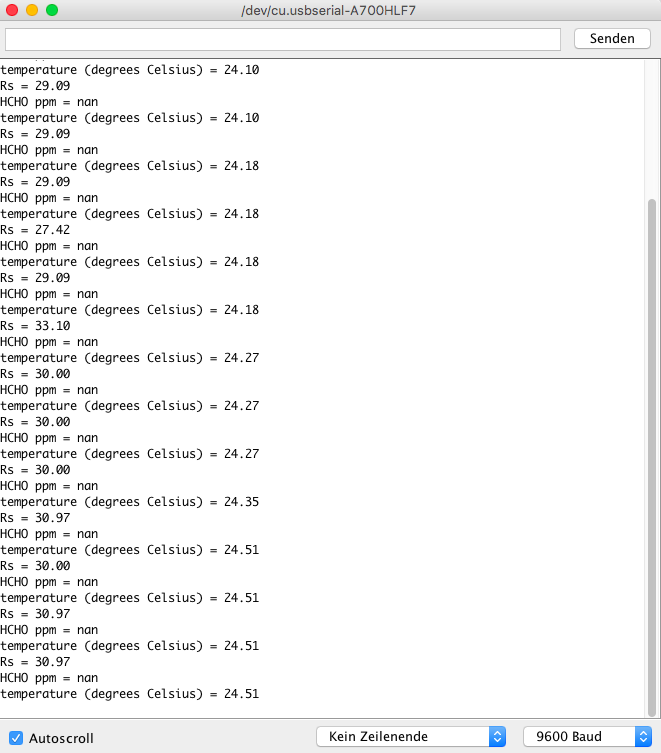3. Dust sensor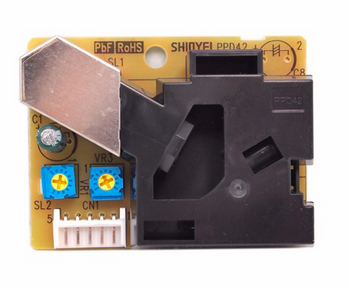In the following you can find the data reading code for dust sensor from the seedsstudio.com .

```int pin = 8;
unsigned long duration;
unsigned long starttime;
unsigned long sampletime_ms = 30000;//sampe 30s ;
unsigned long lowpulseoccupancy = 0;
float ratio = 0;
float concentration = 0;

void setup()
{
Serial.begin(9600);
pinMode(pin,INPUT);
starttime = millis();//get the current time;
}

void loop()
{
duration = pulseIn(pin, LOW);
lowpulseoccupancy = lowpulseoccupancy+duration;

if ((millis()-starttime) > sampletime_ms)//if the sampel time == 30s
{
ratio = lowpulseoccupancy/(sampletime_ms*10.0);  // Integer percentage 0=>100
concentration = 1.1*pow(ratio,3)-3.8*pow(ratio,2)+520*ratio+0.62; // using spec sheet curve
Serial.print(lowpulseoccupancy);
Serial.print(",");
Serial.print(ratio);
Serial.print(",");
Serial.println(concentration);
lowpulseoccupancy = 0;
starttime = millis();
}
}
```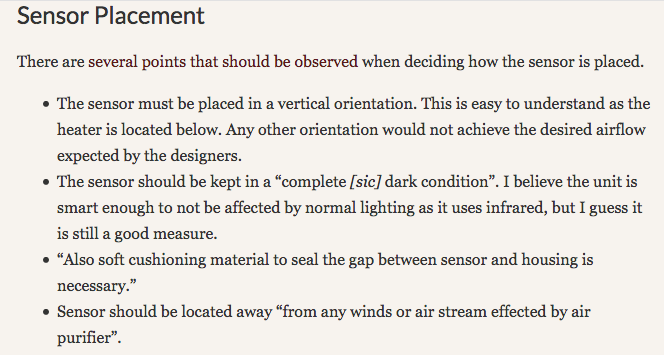4. Stepper Motor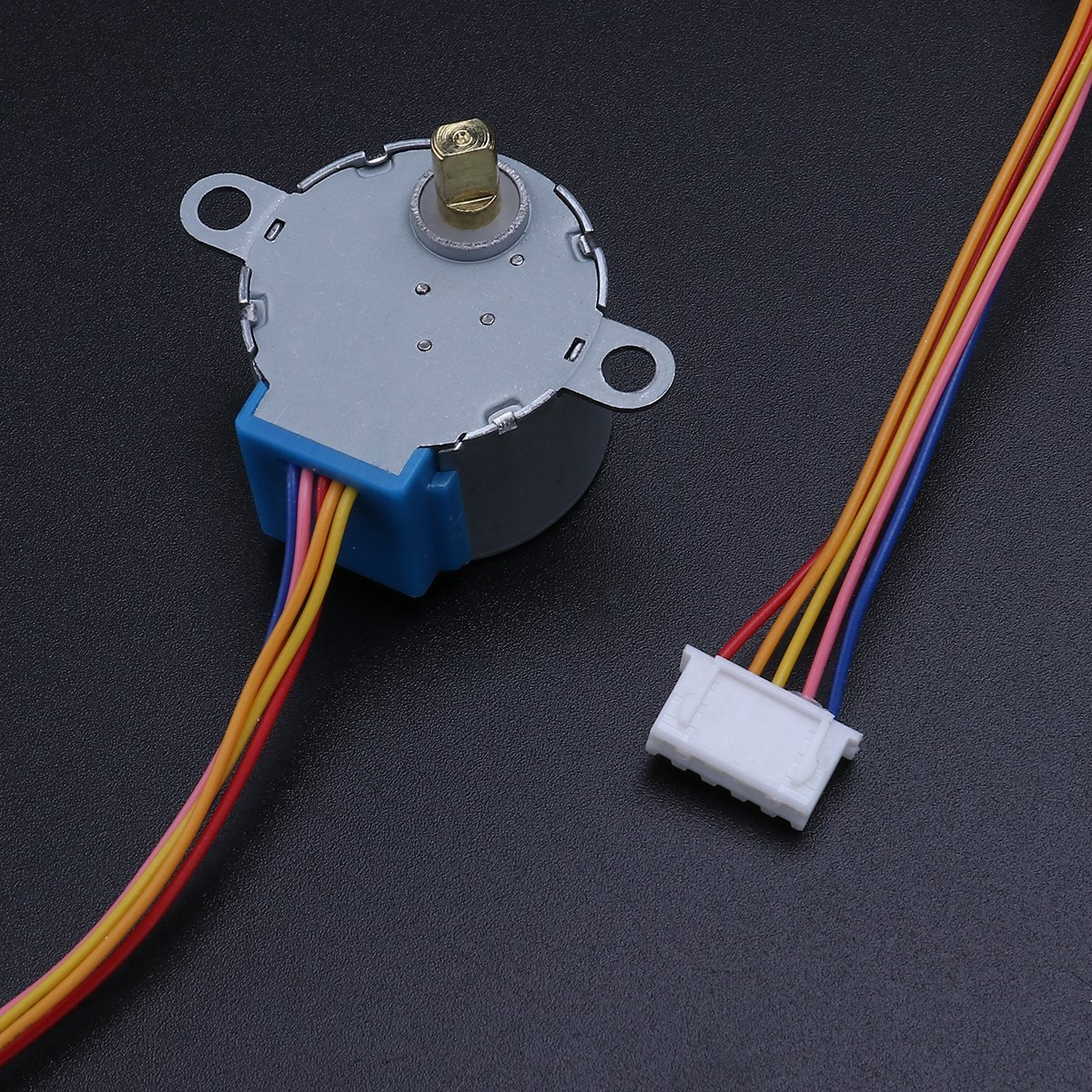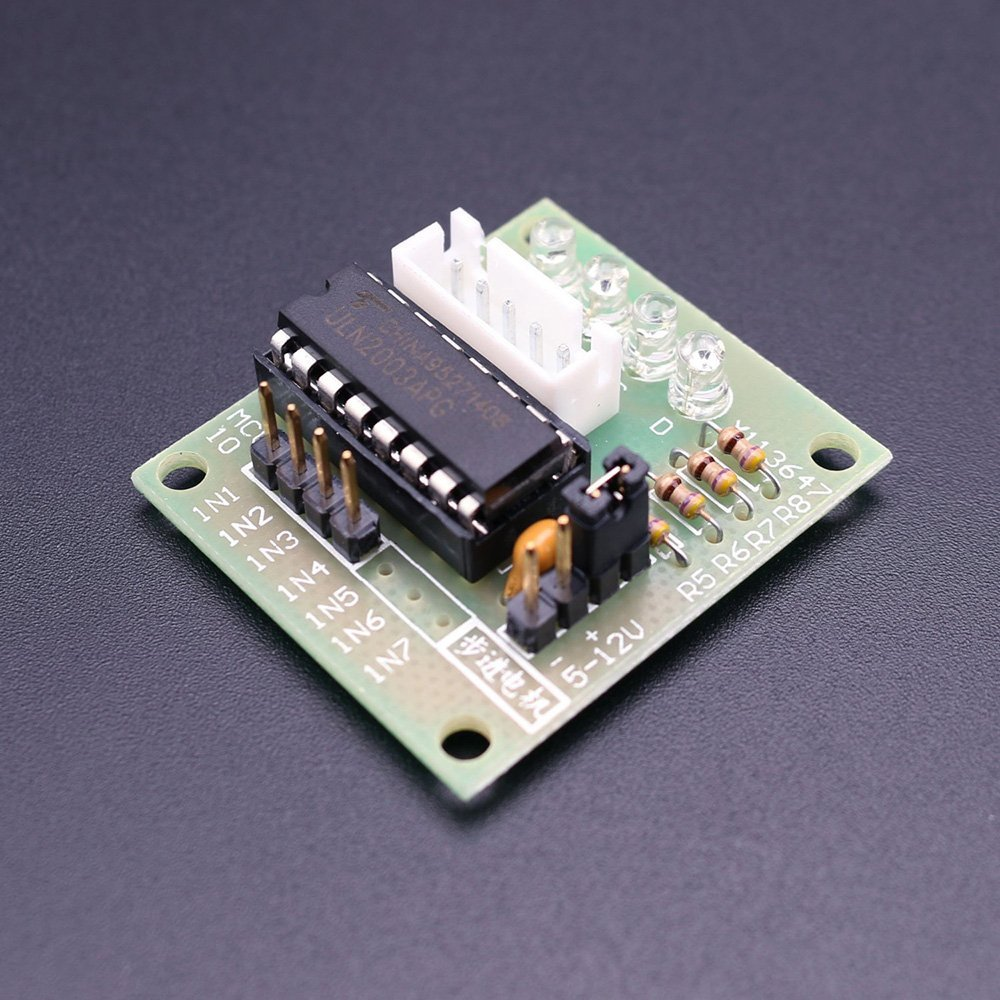Simple stepper motor code:
```    /*
Stepper Motor Demonstration 1
Stepper-Demo1.ino
Demonstrates 28BYJ-48 Unipolar Stepper with ULN2003 Driver
Uses Arduino Stepper Library

DroneBot Workshop 2018
https://dronebotworkshop.com
*/

//Include the Arduino Stepper Library
#include

// Define Constants

// Number of steps per internal motor revolution
const float STEPS_PER_REV = 32;

//  Amount of Gear Reduction
const float GEAR_RED = 64;

// Number of steps per geared output rotation
const float STEPS_PER_OUT_REV = STEPS_PER_REV * GEAR_RED;

// Define Variables

// Number of Steps Required
int StepsRequired;

// Create Instance of Stepper Class
// Specify Pins used for motor coils
// The pins used are 8,9,10,11
// Connected to ULN2003 Motor Driver In1, In2, In3, In4
// Pins entered in sequence 1-3-2-4 for proper step sequencing

Stepper steppermotor(STEPS_PER_REV, 10, 8, 9, 7);

void setup()
{
// Nothing for stepper  (Stepper Library sets pins as outputs)
}

void loop()
{

// Rotate CW 1/2 turn slowly
StepsRequired  =  2*STEPS_PER_OUT_REV  ;
steppermotor.setSpeed(400);
steppermotor.step(StepsRequired);
delay(1000);

// Rotate CCW 1/2 turn quickly
StepsRequired  =  - 2* STEPS_PER_OUT_REV  ;
steppermotor.setSpeed(700);
steppermotor.step(StepsRequired);
delay(2000);

}```
5. RGB LED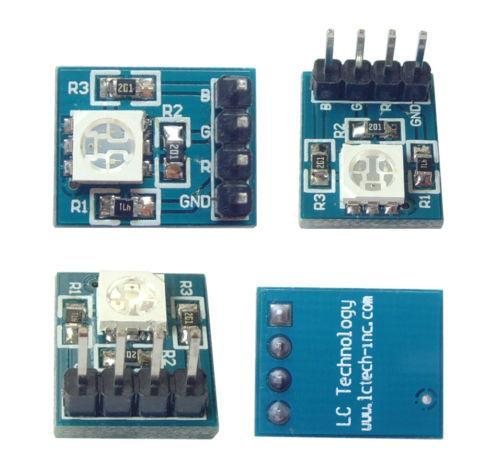Here is a simple RGB LED testing code for Arduino IDE:
```int LEDred = PD5;         // Colour  red is connected on Pin PB2
//int LEDgreen = PD3;     // Colour blue is connected on Pin PB1 (PWM)
int LEDblue = PD6;        // Colour gruen an Pin 6

int p = 3000;             // p is a pause with 3000ms (3 seconds)

int brightness1a = 200;   // Value zwibetween  0 und 255 gives the information about the Brightness of each colour
int brightness1b = 200;
int brightness1c = 200;

int dark = 0;             // Value 0 means Voltage 0V Spannung (LED off).

void setup()
{

pinMode(LEDblue, OUTPUT);
pinMode(LEDgreen OUTPUT);
pinMode(LEDred, OUTPUT);

}

void loop()

{

analogWrite(LEDred, dark);            // now the red LED will be turned off
analogWrite(LEDgreen, brightness1b); // green LED on
delay(p);

analogWrite(LEDgreen, dark);        // green LED off
analogWrite(LEDblue, brightness1c);   //  blue LED on
delay(p);

analogWrite(LEDblue, dark);         // blue LED off
analogWrite(LEDred, brightness1a);
delay(p);

}```